2012款 福克斯两厢 1.6L 自动 舒适型

(新车裸车13万+购置税1.11万)

A级
B级
C级
D级
E级

 汽车品牌： 福克斯 上牌时间 2015-06 表显里程： 5.1万公里 颜色： 白色车身黑色内饰 所在地： 郑州 排放标准： 国4 发动机： 1.6L 变速箱： 初检日期： 2015-03-02 使用性质： 非运营 车源编号： bj_20200910002

浏览388152项车况鉴定结果

• 排除事故车
• 前横梁
• 水箱框架
• 右前纵梁
• 右前翼子板内骨架
• 右前减震器座
• 右侧A柱
• 右侧B柱
• 右侧C柱
• 右后翼子板内骨架
• 右后减震器座
• 后备箱底板
• 后横梁
• 右后纵梁
• 左后纵梁
• 左后减震器座
• 左后翼子板内骨架
• 左侧C柱
• 左侧B柱
• 左侧A柱
• 左前纵梁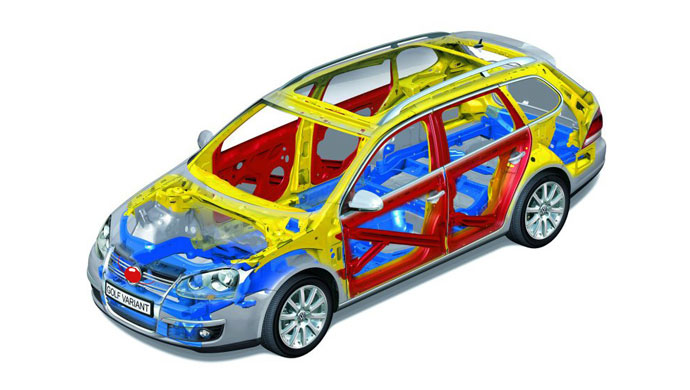• 排除火烧车
• 车上线束和橡胶制品
• 发动机舱及隔夹层
• 车辆覆盖件
• 排除水淹车
• 安全带根部
• 座椅滑轨及内套绒布
• 中控台内线束及插头
• 发动机舱内零部件及室内底板
• 手续合法性检查
• 非抵押
• 非盗抢
• 非走私
• 车身外观及附属件
• 右前翼子板
• 右前门
• 右后门
• 右后翼子板
• 后备箱盖
• 后保险杠
• 左后翼子板
• 左后门
• 左前门
• 左前翼子板
• 车顶
• 引擎盖
• 前保险杠
• 前后挡风玻璃
• 四门车窗玻璃
• 前大灯
• 雾灯
• 后尾灯
• 刹车灯
• 转向灯
• 倒车灯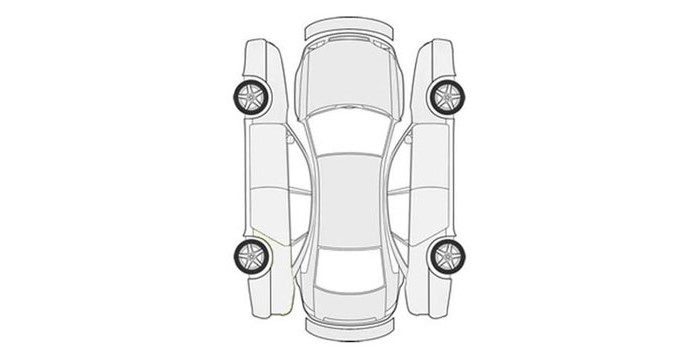• 刹车系统
• 刹车片
• 刹车卡钳
• 刹车分泵
• 制动油管
• ABS泵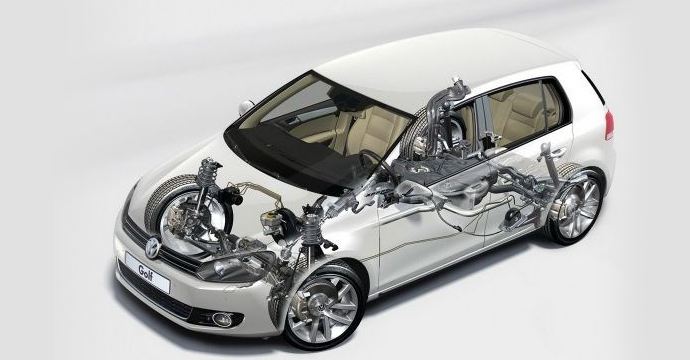• 行驶系统
• 减震器
• 减震悬挂
• 安装缓冲块
• 支臂
• 球头
• 防尘罩
• 平衡杆
• 轮胎花纹≥1.6mm
• 轮毂
• 转向系统
• 转向拉杆
• 球头
• 转向机
• 转向助力液压管路
• 传动系统
• 驱动半轴
• 球笼
• 传动轴
• 万向节
• 驱动桥
• 差速器和分动器
• 下护板
• 燃油管路
• 燃油箱
• 排气管
• 内饰及电子设备
• 遥控钥匙
• 电控开关
• 中控台
• 方向盘及附属设备
• 安全气囊
• 空调
• 多媒体系统
• 导航倒影
• 车身雷达
• 天窗
• 雨刷
• 挡把
• 车内座椅
• 顶棚内衬
• 车门内衬及拉手
• 油门及刹车踏板
• 底板
• 后备箱
• 备胎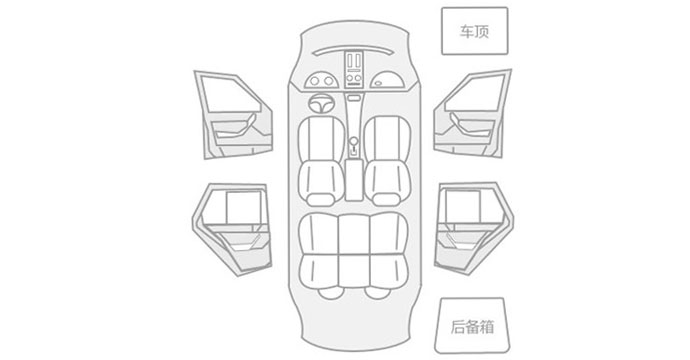• 发动机舱
• 制动总泵
• 制动真空助力泵
• 软管
• 刹车油
• 机油
• 防冻液
• 转向助力油
• 转向助力泵
• 点火线圈
• 火花塞
• 燃油压力
• 喷油嘴
• 变速箱和发动机
• 水泵
• 冷凝器
• 散热器
• 风扇
• 节气门
• 进气管道
• 皮带
• 涨紧轮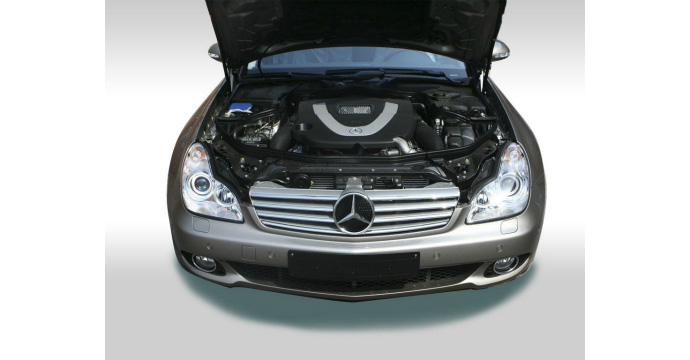• 启动
• 发动机
• 减速
• 发动机
• 变速箱
• 传动系统
• 行驶系统
• 转向系统
• 制动系统
• 制动
• 制动系统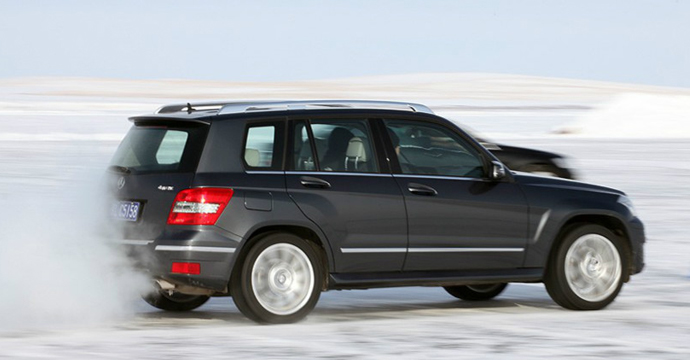• 起步
• 发动机
• 变速箱
• 传动系统
• 行驶系统
• 匀速
• 发动机
• 变速箱
• 传动系统
• 行驶系统
• 转向系统
• 加速
• 发动机
• 变速箱
• 行驶系统
• 传动系统

1.由于初检场地不具备举升机设备，部分车辆的底盘情况无法现场查看。2.部分车辆由于场地限制不便挪动，无法路测。但为了确保车况精准无误，在您定车后，我们还会提车到店再次进行包括底盘，路测在内的全面车况复检，确保万无一失。